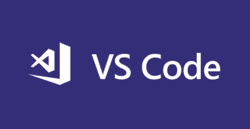```#include <iostream>
using namespace std;

struct hashing
{
int value;
int key;
};

void put(int value, hashing hash[],int n) {
hash[value % n].value = value;
hash[value % n].key = (value % n);
}

int get(int key, hashing hash[]) {
return hash[key].value;
}

int main()
{
int n;

struct hashing hash[n];
cin >> n;
for (int t=0;t<n;t++) {
put(t+1,hash,n);
cout << "Inserted : " << (t+1) << endl;
}
int temp;
cin >> temp;
cout << get(temp,hash) << endl;
}```
```double AttackerSuccessProbability(double q, int z)
{
double p = 1.0 - q;
double lambda = z * (q / p);
double sum = 1.0;
int i, k;
for (k = 0; k <= z; k++)
{
double poisson = exp(-lambda);
for (i = 1; i <= k; i++)
poisson *= lambda / i;
sum -= poisson * (1 - pow(q / p, z - k));
}
return sum;
}```
bookmark
dashboard

Fri Jan 03 2020 19:00:00 GMT+0000 (UTC) https://github.com/manosriram/Data-Structures/blob/master/Hashing/basicHash.cpp

#ios #swift #apps #hash #security
bookmark
dashboard

Sun Dec 29 2019 20:10:36 GMT+0000 (UTC)

#commandline #interesting #cyberattacks #security #unix
bookmark
dashboard

Thu Dec 26 2019 16:59:22 GMT+0000 (UTC)

#C #C++ #historicalcode #interesting #cyberattacks #security

#### Save snippets that work with our extensions